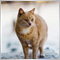• Visão global
• Comentários (2)
• Discussão (6)

# Channel Builder

The Channel Builder (CB) or Ivanov Bands indicator is a broad generalization of the Bollinger Bands indicator. First, in CB, the mean line <X> is calculated using various averaging algorithms. Secondly, the mean deviations calculated by Kolmogorov averaging are plotted on both sides of the middle line <X>.

The middle line <X>, besides the standard SMA, EMA, SMMA and LWMA averaging algorithms, can be Median = (Max + Min) / 2 sliding median (which is the default). In addition, for the calculation of <X>, the moving averaging algorithms <XF> (fast) and <XS> (slow) are used, which are developed by the author. These algorithms specifically filter out the harmful noise that is largely present in the highly volatile currency market. The filtering algorithm <XF> allows you to quickly identify the beginning of the trend.  And the filtering algorithm <XS> allows you to better define the transition to flat.  And, finally, CB allows you to build a weighted by the volume averaging of the price <XV>.

CB allows you to plot an infinite number of different channels similar to Bollinger Bands, but different from it, including Bollinger Bands only as a special case. CB is essentially an experimental stand form plotting and studying different channels in order to find the most suitable one for the manual or automatic strategy used by trader.

CB allows (through various filtering) (1) determine the earliest moment of transition from flat to trend or vice versa, (2) from trend to flat, and when building channels, you can (3) level out the sharp price spikes (that is, the robust method), obtaining smooth lines (with weak response to spikes) for more reliable channels, or, conversely, (4) make the channels very sensitive to such spikes, which allows using sharp breaks of their lines as very reliable sources of additional information on changes in the price movement direction.

The CB indicator uses six different particular implementations of the general algorithm for calculating the average deviation from <X>, while only the first of them - (1) mean power deviation leads to construction of the Bollinger Bands lines in the case of exponent n=2  and <X>=SMA.  In addition, CB also employs algorithms for calculating the following averages: (2) logarithmic, (3) exponential, (4) exponential root mean square, (5) sinusoidal and (6) angular deviations. Each of them (except for logarithmic) can be configured to robust and inverse modes.

The formulas for moving averages and mean deviations used to construct the CB channels are presented in the last screenshots.

### Setting up the indicator

• Price type - applied price. Values: Close price (default), Open price, High price, Low price, Median price ((high + low)/2), Typical price ((high + low + close)/3), Weighted price ((high + low + 2*close)/4).
• The averaging method - averaging method. Values: Simple, Exponential, Smoothed, Linear weighted, Median=(Max+Min)/2 (default), <XF>, <XS> and  <XV>.
• Choose simulation Bands mode – select the algorithm for calculating the mean deviations. Values:
• 1. (<(|x - <x>|)^n>)^(1/n) - mean power (default)
• 2. exp(<ln(|x - <x>|)>) - mean log
• 3. n*std*ln(<exp(|x - <x>|/(n*std))>) - average exponential
• 4. n*std*sqrt(ln(<exp(sign(n)*((x - <x>)/(n*std*))^2)>)) – exponential root mean square
• 5. n*std*arcsin(<sin(pi*|x - <x>|/|n*std|/2)>)/(pi/2) - average sinusoidal
• 6. n*std*sin(<arcsin(|x - <x>|/|n*std|)>) - angular mean
• The averaging period - averaging period. Any integer (20 by default).
• Channel width factor 'K' - channel width factor. Values: any real number (2.0 by default).
• Exponent 'n' - exponent for (1) the exponential averaging algorithm. Scale multiplier for other averaging types (3-6). Values: any real number except 0 (2.0 by default).2372
2019.11.06 16:55

good8582
2019.03.10 14:51

Thanks for share